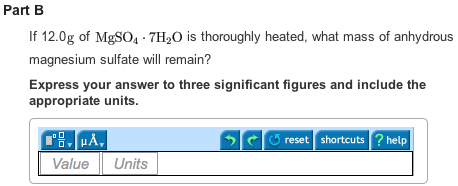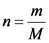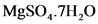# Name the hydrate MgSO4 . 7H2O. If 12.0g of MgSO4 . 7H20 is thoroughly heated, what mass of anhydrous

Name the hydrate MgSO4.7H2O?Concepts and reason
The concepts used to solve this problem are the concept of chemical nomenclature as well as the concept of molecular mass. The correct naming of a chemical compound is represented by the chemical nomenclature. The chemical nomenclature represents certain rules to determine the systematic nomenclature and is developed by the IUPAC (International Union of Pure and Applied Chemistry).
The molecular mass (also referred as the molecular weight) represents the sum total of the atomic weight of each constituent part (element), which is multiplied by the number of atoms of that particular element in its molecular formula. The moles of any solute is represented by the given weight of solute per molecular weight of the solute.

Fundamentals
In the case of the nomenclature for the polyatomic substances/compounds (formed by polyatomic ions), two parts should be differentiated first. These parts are cation (positive part) and anion (negative part).
The name of the cation part is written on the left side followed by the name of anion part on the right side. The compounds containing polyatomic ions are written in parenthesis. The number of atoms is mentioned in the subscript.

In the case of the nomenclature for hydrates, two parts of a hydrate are taken into consideration, that is, an anhydrous salt and the water of hydration. The anhydrous salt is named first and the prefix is determined based on the number of the water molecule. The word hydrate is added to the end of the prefix.

The molecular mass is calculated by adding the atomic weight of each constituent part (element), which is multiplied by the number of atoms of that particular element in its molecular formula. The moles of any solute (n) are calculated as follows:Here, m is given weight and M is molecular weight.

Part A
The correct nomenclature for a hydrateis Magnesium sulfate heptahydrate.

Part B
Upon heating 12g of,5.78 g of anhydrous magnesium sulfate will remain.